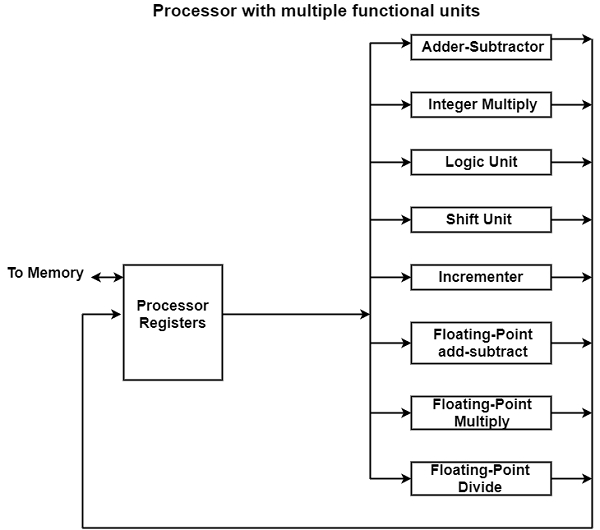# What is Parallel Processing?

Parallel processing is an approach that can denote a huge class of methods that can give simultaneous data-processing functions to improve the computational speed of a computer system.

Rather than processing each instruction sequentially as in a traditional computer, a parallel processing system can implement concurrent data processing to manage quicker implementation time.

For example, while an instruction is being implemented in the ALU, the next instruction can be read from memory. The system can have multiple ALUs and can implement various instructions at a similar time.

The system can have multiple processors operating together. Parallel processing aims to speed up the computer processing efficiency and raised its throughput, that is, the amount of processing that can be accomplished during a given interruption of time.

The number of hardware increases with parallel processing and with it, the value of the system improves. But, technological developments have decreased hardware costs to the point where parallel processing methods are economically possible.

Parallel processing can be considered from multiple levels of complexity. At the lowest level, we categorize parallel and serial operations by the method of registers used. Shift registers perform in serial fashion one bit at a time, while registers with parallel load function with all the bits of the word together.

Parallel processing at a greater level of complexity can be managed by having a multiplicity of functional units that implement identical or various operations together.

Parallel processing is created by assigning the information among the several functional units. For example, the arithmetic, logic, and shift operations can be separated into three units and the operands diverted to each unit under the supervision of a control unit.

The figure displays one possible method of breaking the implementation unit into eight functional units performing in parallel. The operands in the registers are used to one of the units relies upon the operation particularized by the instruction-related with the operands.

The operation implemented in each functional unit is denoted in each block of the diagram. The adder and integer multiplier implements the arithmetic operations with integer numbers.The floating-point operations are divided into three circuits working in parallel. The logic, shift, and increment operations can be implemented together on multiple data.

All units are autonomous of each other, therefore one number can be transferred while another number is being incremented. A multifunctional organization is generally associated with a complex control unit to equivalent all the activities among the several components.